• 求一元二次方程的跟 import java.util.*; public class Example2{  public static void main(String[] args) {   double a,b,c;   double p,q;   double x1,x2;   ...
求一元二次方程的跟
import java.util.*;
public class Example2{
public static void main(String[] args) {
double a,b,c;
double p,q;
double x1,x2;
System.out.println("请输入一元二次方程的三个系数：");
p=-b/(2*a);
q=Math.sqrt(b*b-4*a*c)/(2*a);
x1=p+q;
x2=p-q;
System.out.println("一元二次方程的跟是：");
System.out.printf("x1=%.2f,x2=%.2f\n",x1,x2);
}
}



展开全文• java求一元二次方程的根关注:168答案:2mip版解决时间 2021-02-03 01:41提问者青春统帅2021-02-02 10:42out;+k);if(p&gt.in);方程只有一个跟"System.nextDouble()import java.println(&quot.sqrt(p))/.out....
java求一元二次方程的根关注:168  答案:2  mip版解决时间 2021-02-03 01:41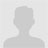提问者青春统帅2021-02-02 10:42out;+k);if(p&gt.in);方程只有一个跟"System.nextDouble()import java.println(&quot.sqrt(p))/.out.println ("if (a;(2*a).util.nextDouble();}}else{System;System.out.println("=0){if(p>double b=sc;x2="0){double x1=(-b+Math;无解"double c=sc.out;double a=sc;public class a1{public void main(String args[]){Scanner sc=new Scanner(System;double x2=(-b-Math;(2*a).println("x1="System;+x1)!=0){double p=b*b-4*a*c;);+x2).nextDouble().sqrt(p))/(2*a).*;}else{double k=-b/运行为什么有这个错误？最佳答案二级知识专家戒烟戒酒戒粗口2021-02-02 12:12public void main(String args[]){这句应改为public static void main(String[] args){养成好习惯！全部回答1楼假装安慰2021-02-02 13:42程序中您自己写的那个 sqrt 方法，返回值都是 0，那怎么行呢。。开平方的话直接用 math.sqrt()修改如下：import java.util.scanner;import java.lang.math;public class shiyan {public static void main(string[] args) {double s=0,x1,x2;system.out.println("输入系数 a b c: ");scanner in=new scanner(system.in);double a=in.nextint();double b=in.nextint();double c=in.nextint();s = b*b-4*a*c;if(a==0){system.out.println("二次方程系数a不能为0！");return;}else if(s==0){x1 = -b/(2*a);system.out.println("x1="+x1);return;}else if(s>0){double t = math.sqrt(s);x1 = (-b+t)/(2*a);x2 = (-b-t)/(2*a);system.out.println("x1="+x1);system.out.println("x2="+x2);return;}else if(s<0){system.out.println("该一元二次方程没有实根");return;}}}我要举报如以上问答内容为低俗/色情/暴力/不良/侵权的信息，可以点下面链接进行举报，我们会做出相应处理，感谢你的支持！→点此我要举报以上信息！←推荐资讯大家都在看
展开全文• 求一元二次方程的根。 【问题描述】编写程序，从键盘输入三个系数ax2+bx+c=0，计算方程的解并输出。需要考虑方程有虚根、方程有实根、方程是一元一次方程、没有根等。输出方程的解并保留6位小数。 import java....
求一元二次方程的根。
【问题描述】编写程序，从键盘输入三个系数ax2+bx+c=0，计算方程的解并输出。需要考虑方程有虚根、方程有实根、方程是一元一次方程、没有根等。输出方程的解并保留6位小数。
import java.text.DecimalFormat;
import java.util.*;
public class  Equation{
public static void main(String[] args) {
System.out.println("请输入方程的系数a、b、c：");
int a, b, c;
DecimalFormat df = new DecimalFormat("0.000000");
int delta;
double x1, x2;
delta = b * b - 4 * a * c;
if (a == 0 && b == 0 && c == 0) {
System.out.println("方程有无限根。");
return;
}
if (a == 0 && b == 0 & c != 0) {
System.out.println("方程无解。");
return;
}
if (delta == 0) {
x1 = b / -2.0 / a;
x2 = b / -2.0 / a;
System.out.println("方程有两个相同实根, x1 =  "
+ df.format(x1) + ",  x2 = " + df.format(x2));
}
if (delta > 0) {
x1 = (-b + Math.sqrt(delta)) / 2.0 / a;
x2 = (-b - Math.sqrt(delta)) / 2.0 / a;
System.out.println("方程有两个不同实根, x1 = "
+ df.format(x1) + ",  x2 = " + df.format(x2));
}
if (delta < 0) {
double s, x;
s = -b / 2.0 / a;
x = Math.sqrt(-delta) / 2.0 / a;
System.out.println("方程有虚根, x1 = "
+ df.format(s) + " + " + df.format(x) + "i,  x2 = "
+ df.format(s) + " - " + df.format(x) + "i");
}
}
}

运行结果：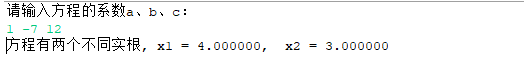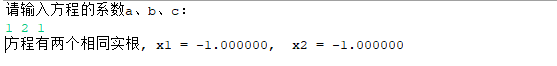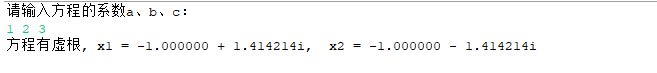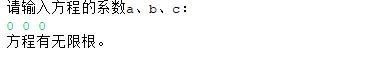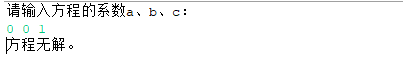展开全文• 一元二次方程ax2+bx+c=0，输入a,b,c三个系数，求解方程，结果有三种情况：两个实数根、一个.../*** 计算一元二次方程的根* @author TongQiang**/public class Equation {double a,b,c; //方程的系数double x1,x2; /...
一元二次方程ax2+bx+c=0，输入a,b,c三个系数，求解方程，结果有三种情况：两个实数根、一个实数根、两个复数根。/*** Equation.java*/package cn.edu.uibe.oop;/*** 计算一元二次方程的根* @author TongQiang**/public class Equation {double a,b,c; //方程的系数double x1,x2; //两个根double r;     //实部double v;     //虚部int type;     //0表示一个根，1表示两个实根，2表示两个复数的根public Equation(double a,double b,double c){this.a = a;this.b = b;this.c = c;}private double delta(){return b*b-4*a*c;}public void calculate(){double d = delta();if(Math.abs(d) < 1E-5){type = 0;x1 = -b/(2*a);x2 = x1;}else if(d > 0){type = 1;x1 = (-b+Math.sqrt(d))/(2*a);x2 = (-b-Math.sqrt(d))/(2*a);}else{type = 2;r =  -b/(2*a);v = Math.sqrt(-d)/(2*a);}}public void showResult(){switch(type){case 0:System.out.println("方程只有一个实根，x1=x2="+x1);break;case 1:System.out.println("方程有两个实根，分别是：x1="+x1+"/tx2="+x2);break;case 2:System.out.println("方程有两个复数根，分别是："+r+"+"+v+"i,/t"+r+"-"+v+"i");break;}}public static void main(String[] args) {Equation eq1 = new Equation(1,2,2);eq1.calculate();eq1.showResult();Equation eq2 = new Equation(1,-2,1);eq2.calculate();eq2.showResult();Equation eq3 = new Equation(1,6,5);eq3.calculate();eq3.showResult();}}
展开全文• 提高题 1) 设有一元二次方程如下: aX bx c=0 试根据从键盘输入的 a,b,c 求解方程的根。 【提示】要考虑各种情形:一是 a 为0的情形,方程根为-c/......(理论考试重点内容实践) 一元二次方程白盒测试要求 1) 自备 C ...
• 这是我java实习作业，完全能运行,感觉还可以就顶下啊！
• Java求一元二次方程的根（实验三） Package和import语句的使用 编写Java应用程序求方程的根。使用package语句将方程的属性即计算根的方法封装在一个有包名的类中，包名是tom.jiafei，类的名字是SquareEquation。编写...
• //创建一个test包import java.util.Scanner;//导入java.util包中Scanner类(使用...//导入java.math包中BigDecimal(用来对超过16位有效位数进行精确运算)//对一元二次方程中各系数不同情况作讨论，列出不同...
• 一元二次方程ax2+bx+c=0，输入a,b,c三个系数，求解方程，结果有三种情况：两个实数根、一个.../*** 计算一元二次方程的根* @author TongQiang**/public class Equation {double a,b,c; //方程的系数double x1,x2; /...
• 求一元二次方程 ax2+bx+c=0ax2+bx+c=0ax^2+bx+c=0 的根，分情况讨论，结果保留2位小数。 02. 一元二次方程ax2+bx+c=0ax2+bx+c=0ax^2+bx+c=0 的根 由代数基本定理，一元二次方程有且仅有两个根（重根按重数计算...
• Java语言求一元二次方程的两个实的程序的编写，要有完整的代码和相关的注释说明。开发语言
•  编制一个java application应用程序，3X2+4X-1=0的根 该程序用到一个Math.sqrt（）函数根号； 程序如下： 运行结果：
• 使用Java编写一个程序实现一元二次方程的求根，用户可以自行输入一元二次方程各个参数的值，并通过该程序进行求根。在编写该程序之前首先需要学会编写一个“应声虫”程序，并在此基础上实现参数的手动输入。 2、...
• } private double calculateValidDisk() { if (a == 0) { throw new EquationException("不是二次方程", EquationException.NONE_EQUATION); } double disk = b * b - 4 * a * c; if (disk ) { throw new ...
• import java.util.Scanner; public class Main { ... 求一元二次方程的根 */ public static void main(String[] args) { Scanner s = new Scanner(System.in); double a = s.nextDouble(); double b =pat
• ## Python一元二次方程求根

万次阅读 多人点赞 2018-09-18 17:36:34
在之前博客中我分享了使用Java进行一元二次方程求根的方法，在学习了Python之后我也想使用Python编写一个类似程序，故在编写成功后将该任务分享出来。 2、任务代码 学习过Java和Python人都知道，Python...Python
• 共回答了21个问题采纳率：95.2%import java.util.Scanner;//这个是导入java包,因为你在这后面中用...方程的解不见得全是int吧,用double更准确Scanner scanner = new Scanner(System.in); //第一个Scanner是类名系统...
• 0，这个时候就产生虚根了，写起来比较麻烦，算法是这样举个简单例子说明吧:求一元二次方程:x^2+x+1=0虚根解:△=1^2-4×1×1=-3由求公式x=[-b±√(-△)i]/2a=[-1±√(-△)i]/2=[-1±√3i]/23、NAN这个我写一下...
• 我在2018年5月21日写过一篇文章《使用Java实现一元二次方程求根计算器》，但是该计算器对于一元二次方程的计算只局限于整型变量，对于带有小数的一元二次方程就无计可施了，所以今天就对它进行改进。 2、程序代码...
• 提高题 1) 设有一元二次方程如下: 2 aX bx c=0 试根据从键盘输入的 a,b,c 求解方程的根。 【提示】要考虑各种情形:一是 a 为0的情形,方程根为-c/......Java 复习题汇总 编程题 1、编程序,输入 a,b,c,方程 package ...
• 求一元二次方程:ax2+bx+c=0的根，a、b、c由键盘输入。算法设计与分析
• * 程序版权和版本声明部分* Copyright (c) 2012, 烟台大学计算机学院学生* All rights reserved.* 作 者： 刘镇* 完成日期： 2012 年 11 月 23 日* 版 本 号： 2.014* 对任务及求解方法描述部分* 问题描述：编写...
• * 程序版权和版本声明部分* Copyright (c) 2012, 烟台大学计算机学院学生* All rights reserved.* 作 者： 刘镇* 完成日期： 2012 年 11 月 23 日* 版 本 号： 2.014* 对任务及求解方法描述部分* 问题描述：编写...
• * 程序版權和版本聲明部分* Copyright (c) 2012, 煙台大學計算機學院學生* All rights reserved.* 作 者： 劉鎮* 完成日期： 2012 年 11 月 23 日* 版 本 號： 2.014* 對任務及求解方法描述部分* 問題描述：編寫...
• * 输入一元二次方程系数， * 若有实数求根并输出， * 否则输出“不是二次方程或没有实数信息。 * @author Administrator * */ public class T3_3 { public static void main(String[] args) { ...
• //求一元二次方程a*x*x+b*x+c = 0 的根， a,b,c的数据从键盘输入 import java.util.*; public class Example3_6{ public static void main(String[] args){ double a , b , c; double p , q , disc; double ...
• 高分悬赏：利用Java语言求一元二次方程的两个实数，程序代码要首先判断是否存在实开发语言# 求一元二次方程的根javajava 订阅